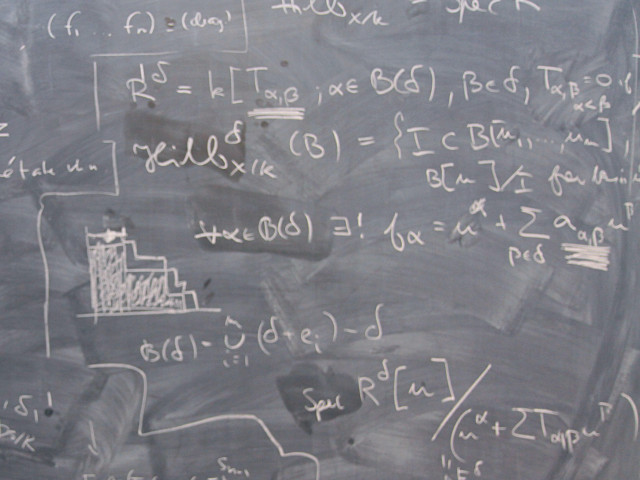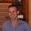Basic course in calculus in several variables with applications.

Course offering missing for current semester as well as for previous and coming semesters

## Content and learning outcomes

### Course contents

Functions of several variables. Basic topological concepts in Euclidean space. Differentiability and linear approximation of mappings.

The chain rule in general form. Implicit and inverse function theorems.

Extremal problems with and without constraints. Multiple integrals, change of variables, geometric applications. Elementary vector analysis: Curve and surface integrals, the integral formulas of Gauss, Green and Stokes.

### Intended learning outcomes

Having passed the course examination, the student is expected to

• Be able to formulate the concept of functions in several variables, including domain of definition, range, composition of functions, inverse functions, level curves and surfaces, and, in simpler cases, the concepts open, closed, bounded sets and boundary of a set.
• Know how to calculate partial derivatives in several variables, and that when the derivatives are continuous, the order of differentiation does not matter. Be in command of the chain rule and know how to transform elementary differential expressions to new coordinates.
• Know how to use the second derivatives to characterise critical points, principally in two dimensions.
• Know how to determine greatest and smallest values for continuous functions on closed and bounded sets. In simpler cases be able to use Lagrange's method to optimise functions under constraints.
• Know how to find equations for tangent planes. Know how to calculate the gradient of a function, and its interpretation as the normal to tangent lines and planes respectively. Know how to calculate directional derivatives.
• Be able to use linear approximation and Taylor's formula, principally in two dimensions and of order two.
• Know how to calculate the curvature for curves in dimension two and three.
• Know how double integrals arise as limits of (double)  Riemann sums. Know how to calculate double, and in simpler cases, triple integrals, by iterated integration. This  includes determining  limits of integration in the successive integrations.
• Be able to use multiple integrals in applications, e g to determine areas and volumes.
• Be able to calculate curve integrals i two and three dimensions,  and surface integrals in three dimensions. Know how to use, in elementary situations,  Green's formula and the  divergence theorem.
• Know how to change path in curve integrals and in simpler cases determine wether a potential function exists and in the affirmative case find it.

For higher marks the student shall also:

• Generally be able to solve more difficult and complex problems and show a higher degree of insight in theory and concepts, particularly the theory of continuous functions.

• Know the definitions of limit and continuity and be able to prove that given functions are continuous. Know the difference between these concepts in one and in higher dimension.

• Be able to define differentiability and know criteria for this to hold.

• Know in dimensions two and three, Taylor's formula of higher order, including studies of the second derivatives at critical points.

• Know how to find derivatives using implicit differentiation of systems of equations.

• Know how to formulate and use of the orientation of curves and surfaces, when curve integrals are independent of path, existence of potential function and phenomena arising in connection with singular fields and potentials.

• Know how to formulate and use the Stokes theorem.

### Course Disposition

No information inserted

## Literature and preparations

### Specific prerequisites

SF1602

Mandatory for first year. Not available to other students.

### Recommended prerequisites

No information inserted

### Equipment

No information inserted

### Literature

No information inserted

## Examination and completion

If the course is discontinued, students may request to be examined during the following two academic years.

A, B, C, D, E, FX, F

### Examination

• TEN1 - Examination, 9,0 hp, betygsskala: A, B, C, D, E, FX, F

Based on recommendation from KTH’s coordinator for disabilities, the examiner will decide how to adapt an examination for students with documented disability.

The examiner may apply another examination format when re-examining individual students.

### Other requirements for final grade

One written exam (TEN1;9 hp).

### Opportunity to complete the requirements via supplementary examination

No information inserted

### Opportunity to raise an approved grade via renewed examination

No information inserted

### ExaminerJonatan Carl Anders Lenells

### Ethical approach

• All members of a group are responsible for the group's work.
• In any assessment, every student shall honestly disclose any help received and sources used.
• In an oral assessment, every student shall be able to present and answer questions about the entire assignment and solution.

## Further information

### Course web

Further information about the course can be found on the Course web at the link below. Information on the Course web will later be moved to this site.

Course web SF1603

SCI/Mathematics

### Main field of study

Mathematics, Technology

First cycle